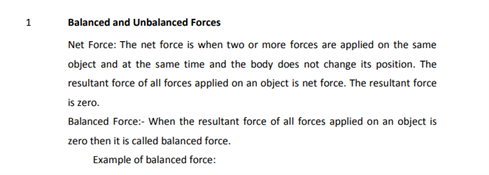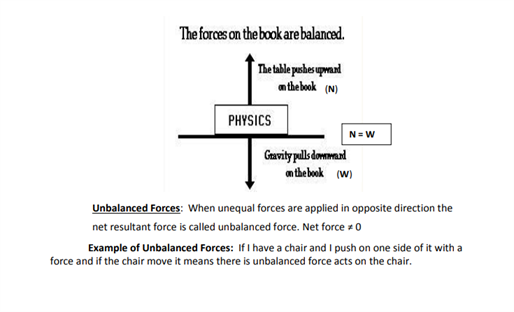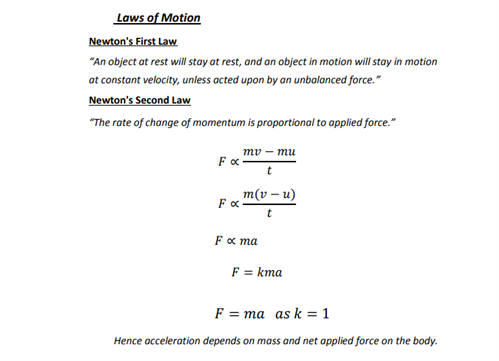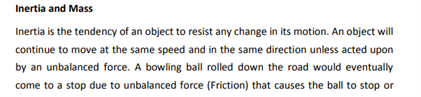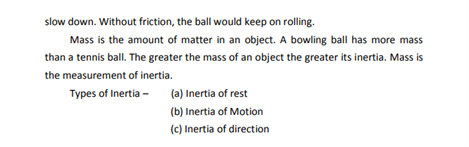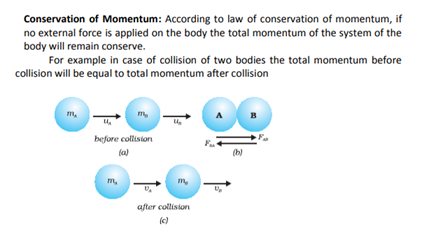# Revision Notes For Class 9 Science Chapter 9 Force and Laws of Motion

Laws of motion is an important law of physics. There are mainly three laws of motion. The First Law of Motion, the Second Law of Motion and the Third Law of Motion. To know that laws of motion students must understand what is a force, there are various kind of forces present in this universe like the balanced and unbalanced force. To master the concepts of this chapter before exam students must revise the concepts. To help students revise the chapter we have given the CBSE revision notes for CBSE class 9 Science chapter 9 force and laws of motion below.